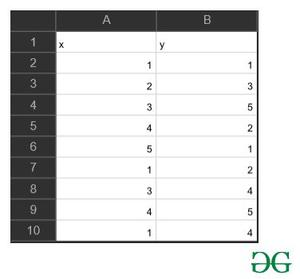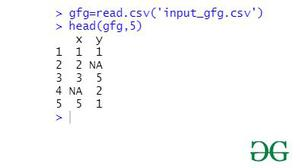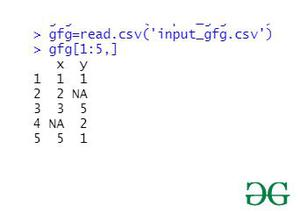# Extract first N rows from dataframe in R

• Last Updated : 26 Mar, 2021

A required number of rows can be retrieved from a dataframe for some computation that demands so. In this article, we will be discussing two different approaches for extracting the first n rows from the data frame

File in Use:This function will help to extract the given first N number of the rows.

Parameters:-

• x:-an object
• n:-a single integer for the first number of elements of x

Returns:-

Returns the first parts of a vector, matrix, table, data frame.

Approach

• Import file
• Pass the number of rows required
• Select rows
• Print selection

Example:

## R

 `gfg=``read.csv``(``'input_gfg.csv'``) ` ` `  `head``(gfg,5)`

Output:Method 2: Using index

Under this method of extracting the first N rows of the data frame, the user must provide the machine with the proper index of the required rows and columns.And with this, it will return the new data frame as per the provided index of rows and columns.

Syntax:

data[row.index, column.index]

Approach

• Import file
• Pass the range of rows to be selected
• Display selection

Example:

## R

 `gfg=``read.csv``(``'input_gfg.csv'``) ` ` `  `gfg[1:5,]`

Output:My Personal Notes arrow_drop_up
Recommended Articles
Page :